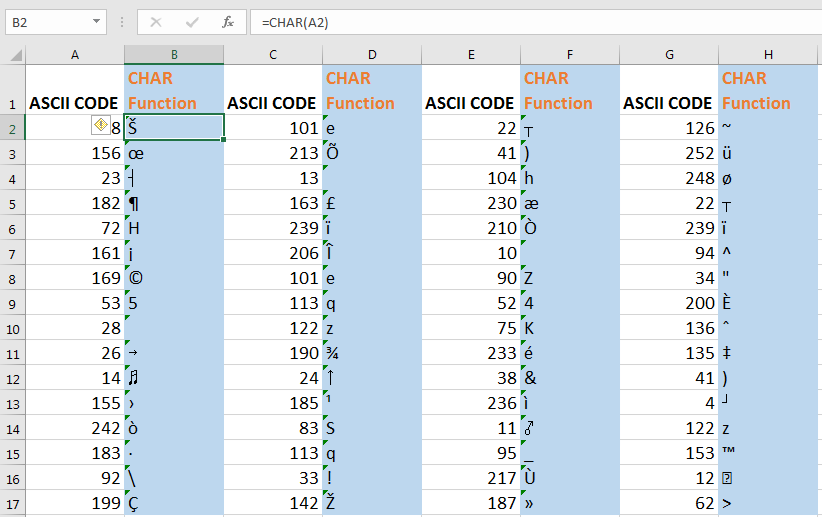# How to use the CHAR Function in Excel | Char

The Excel CHAR function is used for converting a number code into its character. Each character in excel has the number code. To convert code into its equivalent text we use CHAR function.

Syntax

=CHAR(number)

Number: CHAR function only has one parameter and that is the number that you want to convert into text.
Example

If you supply 65 as a parameter to char function it will return A.

=CHAR(65)

I write below formula it will return one.

=CHAR(49)

The CHAR function sees any number as ASCII code. Each ASCII code has its equivalent number or character.

Its fun to see which code returns what character. Here I used rand between to generate random number between 1 and 255 and then applied CHAR function to each number. See what I got. ASCII CODES are 1 to 255 only. Any number above 255 will return #VALUE error.Do you know what is how can you Insert a Line Break using a Formula? Excel CHAR function can help you there. The code for new line is 10 in Microsoft and 13 in excel for MAC.

The counter function of the CHAR function is the CODE function. The CODE function returns the ASCII code of any character supplied to CODE function.

So Yeah guys, this how CHAR function works in excel. Let me know if you have any specific question regarding this function. The comments section is all yours. I will be happy to help you.

Related Article:

Insert a Line Break using a Formula

The CHAR Function in Excel

How to use the UNICHAR Function in Excel

How to use the UNICODE Function in Excel

Popular Articles:

The VLOOKUP Function in Excel

COUNTIF in Excel 2016

How to Use SUMIF Function in Excel

Terms and Conditions of use

The applications/code on this site are distributed as is and without warranties or liability. In no event shall the owner of the copyrights, or the authors of the applications/code be liable for any loss of profit, any problems or any damage resulting from the use or evaluation of the applications/code.